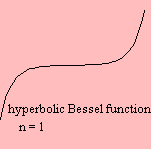# modified Bessel function

## gamma

The modified Bessel function is the solution of the differential equation:

x2 y '' + x y' - (x2 + n2) y = 0

which is slightly different from the Bessel differential equation that defines the Bessel function.

There are two solutions: